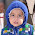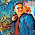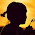## MEANING AND NATURE OF MATHEMATICS

### Meaning of Mathematics

The term ‘Mathematics’ has been interpreted and explained in various ways. This is due to our empirical knowledge and various types of experiences. But ultimately all types of explanations conclusively end at some kinds of relationship with number and space. Thus, mathematics deals with quantitative facts and relationships as-well-as with problems involving space and form.
It enables the man to study various phenomena in space and establish different types of relationship between magnitudes of quantitative and quantitative facts. Therefore it may be concluded that mathematics is the enumerative and calculative part of human life and knowledge the person to given an exact interpretation.

According to New English Dictionary, “Mathematics, in a strict sense, is the abstract science which investigates deductively the conclusions implicit in the elementary conceptions of spatial and numerical relations.”

According to Webster’s Dictionary, “Mathematics is the science of numbers and their operations, interrelations, combinations, generalizations and abstractions and of space configuration and their structure, measurement, transformations and generalizations.”

In the words of Locke, “Mathematics is a way to settle in the mind a habit of reasoning.”

According to Roger Bacon, “Mathematics is the gate and key of the sciences…. Neglect of mathematics work injury to all knowledge, since who is ignorant of it cannot know the other sciences or the things of the world. And what is worse, men who are thus ignorant are unable to perceive their own ignorance and so do not seek a remedy.”

On the basis of above definitions, we can say or conclude that –

### Nature of Mathematics

What is Mathematics and how does it grow are the basic questions which all the students of Mathematics must understand. In school, those subjects which are included in the curriculum must have certain aims and objectives on the basis of which its nature is decided. Mathematics holds a strong and unbreakable position as compared to other school subjects.

With this reason, mathematics is more stable and important than other school subjects. The way in which the structure of a subject becomes weak, its truthfulness, reliability and prediction also decreases in the same manner. On the basis of this specific structure, the nature of each subject is determined and placed in the school curriculum.

It is not necessary that all subjects have same nature. Mathematics has its unique nature thus on the basis of which we can compare it with other subjects. The basis of comparison of two or more subject is their nature. We can understand the nature of Mathematics on the basis of following features –
• Mathematics is a science of space, numbers, magnitude and measurement.
• Mathematics has its own language. Language consists of mathematical terms, mathematical concepts, formulae, theories, principles and signs, etc.
• It gives accurate and reliable knowledge.
• Mathematics knowledge is exact, systematic, logical, and clear so that once it is captured it can never be forgotten.
• Mathematical rules, laws and formulae, are universal and that can be verified at any place and time.
• It develops the ability of induction, deduction and generalisation.
• Mathematics helps in developing scientific attitude among children.
• The study of Mathematics gives the training of scientific method to the children.
• Mathematical knowledge is based on sense organs.
• Mathematics is a systematised, organized and exact branch of science.
• Mathematics involves conversation of abstract concepts into concrete form.
• Mathematics is the science of logical reasoning.
• Mathematics does not leave any doubt in the mind of learner about theories, principles concepts etc.
• Mathematics helps to develop that habit of self-confidence and self reliance in children.
• Mathematics helps in the developments of sense of appreciation among children.
• Mathematics language is well defined, useful and clear.
• It draws numberical inferences on the basis of given information and data.
• Mathematical knowledge is applied in the study of science and in this different branches; for example physics, chemistry, biology, and other sciences.
• It is not only useful for different branches of science but also helps in its progress and organisation.
Thus on the basis of above points we can understand the nature of mathematics and draw conclusion that the structure of mathematics is indeed the basis of its nature and is more strong as compared to other school subjects. That is why its study is essential in school education.

Note: Did you liked the post? Please tell us by your comment below..!!

1.Very good notes

2.Very good notes

3.4.THIS ONE IS THE BEST SITE

5.This one is the best site